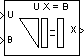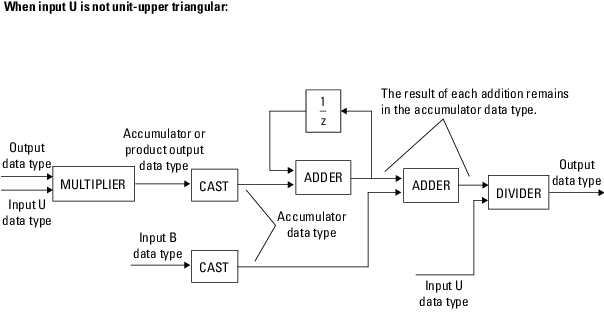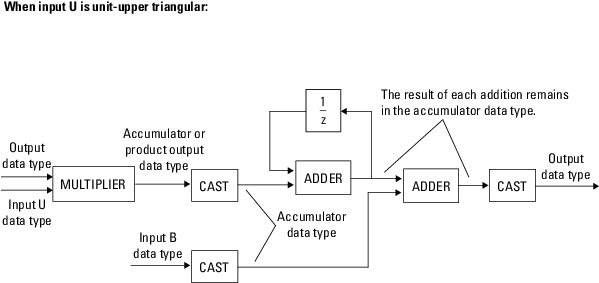Main Content

# Backward Substitution

Solve UX = B for X when U is upper triangular matrix

## Library

Math Functions / Matrices and Linear Algebra / Linear System Solvers

`dspsolvers`

•## Description

The Backward Substitution block solves the linear system UX = B by simple backward substitution of variables, where:

• U is the upper triangular M-by-M matrix input to the U port.

• B is the M-by-N matrix input to the B port.

The M-by-N output matrix X is the solution of the equations. The block does not check the rank of the inputs.

The block uses only the elements in the upper triangle of input U and ignores the lower elements. When you select the Input U is unit-upper triangular check box, the block assumes the elements on the diagonal of U are 1s. This is useful when matrix U is the result of another operation, such as an LDL decomposition, that uses the diagonal elements to represent the D matrix.

The block treats a length-M vector input at port B as an M-by-1 matrix.

### Fixed-Point Data Types

The following diagram shows the data types used within the Backward Substitution block for fixed-point signals.You can set the product output, accumulator, and output data types in the block dialog.

The output of the multiplier is in the product output data type when the input is real. When the input is complex, the result of the multiplication is in the accumulator data type. For details on the complex multiplication performed, see Multiplication Data Types.

## Parameters

Main Tab

Input U is unit-upper triangular

Select this check box only when all elements on the diagonal of U have a value of `1`. When you do so, the block optimizes its behavior by skipping an unnecessary division operation.

Do not select this check box if there are any elements on the diagonal of U that do not have a value of `1`. When you clear the Input U is unit-upper triangular check box, the block always performs the necessary division operation.

Diagonal of complex input U is real

Select to optimize simulation speed when the diagonal elements of complex input U are real. This parameter is only visible when Input U is unit-upper triangular is not selected.

Note

When U is a complex fixed-point signal, you must select either Input U is unit-upper triangular or Diagonal of complex input U is real. When either of these options are selected, the block ignores any imaginary part of the diagonal of U.

Data Types Tab

Note

Floating-point inheritance takes precedence over the data type settings defined on this pane. When inputs are floating point, the block ignores these settings, and all internal data types are floating point.

Rounding mode

Specify the rounding mode for fixed-point operations as one of the following:

• `Floor`

• `Ceiling`

• `Convergent`

• `Nearest`

• `Round`

• `Simplest`

• `Zero`

For more details, see rounding mode.

Saturate on integer overflow

When you select this parameter, the block saturates the result of its fixed-point operation. When you clear this parameter, the block wraps the result of its fixed-point operation. For details on `saturate` and `wrap`, see overflow mode for fixed-point operations.

Product output

Specify the product output data type. See Fixed-Point Data Types and Multiplication Data Types for diagrams showing the use of the product output data type in this block. You can set it to:

• A rule that inherits a data type, for example, `Inherit: Inherit via internal rule`. For more information on this rule, see Inherit via Internal Rule.

• A rule that inherits a data type, for example, `Inherit: Same as first input`.

• An expression that evaluates to a valid data type, for example, `fixdt(1,16,0)`

Click the buttonto display the Data Type Assistant, which helps you set the Product output parameter.

See Specify Data Types Using Data Type Assistant (Simulink) for more information.

Accumulator

Specify the accumulator data type. See Fixed-Point Data Types for illustrations depicting the use of the accumulator data type in this block. You can set this parameter to:

• A rule that inherits a data type, for example, `Inherit: Inherit via internal rule`. For more information on this rule, see Inherit via Internal Rule.

• A rule that inherits a data type, for example, ```Inherit: Same as product output```.

• A rule that inherits a data type, for example, `Inherit: Same as first input`.

• An expression that evaluates to a valid data type, for example, `fixdt(1,16,0)`

Click the buttonto display the Data Type Assistant, which helps you set the Accumulator parameter.

See Specify Data Types Using Data Type Assistant (Simulink) for more information.

Output data type

Specify the output data type. See Fixed-Point Data Types for illustrations depicting the use of the output data type in this block. You can set it to:

• A rule that inherits a data type, for example, `Inherit: Same as first input`

• An expression that evaluates to a valid data type, for example, `fixdt(1,16,0)`

Click the buttonto display the Data Type Assistant, which helps you set the Output data type parameter.

See Control Signal Data Types (Simulink) for more information.

Minimum

Specify the minimum value that the block should output. The default value is `[]` (unspecified). Simulink® uses this value to perform:

• Simulation range checking (see Specify Signal Ranges (Simulink))

• Automatic scaling of fixed-point data types

Maximum

Specify the maximum value that the block should output. The default value is `[]` (unspecified). Simulink uses this value to perform:

• Simulation range checking (see Specify Signal Ranges (Simulink))

• Automatic scaling of fixed-point data types

Lock data type settings against changes by the fixed-point tools

Select this parameter to prevent the fixed-point tools from overriding the data types you specify on the block mask.

## Supported Data Types

PortSupported Data Types

U

• Double-precision floating point

• Single-precision floating point

• Fixed point (signed only)

• 8-, 16-, and 32-bit signed integers

B

• Double-precision floating point

• Single-precision floating point

• Fixed point (signed only)

• 8-, 16-, and 32-bit signed integers

X

• Double-precision floating point

• Single-precision floating point

• Fixed point (signed only)

• 8-, 16-, and 32-bit signed integers

## See Also

### Topics

Introduced before R2006a

## SupportGet trial now